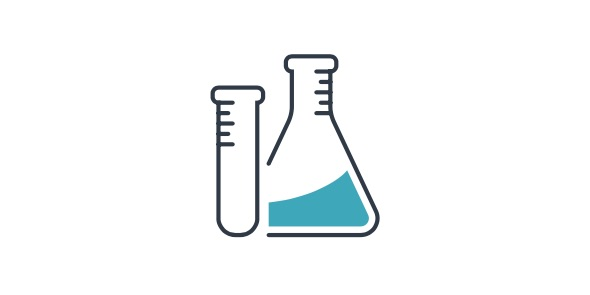# 601stilwell - 6th Grade - Science - Benchmark 1

25 Questions | Total Attempts: 683SettingsCreate your own QuizBenchmarking is the act of comparing something with another based on a particular standard. There are different ways to benchmark when it comes to science in order to get accurate conclusions. How conversant are you with these methods? Take up the simple quiz below and find out. All the best!

• 1.
1. A prediction about a problem that can be tested is(n)______________.
• A.

Hypothesis

• B.

Independent variable

• C.

Dependent variable

• D.

Control

• 2.
2. The factor being measured in an experiment is the _____________.
• A.

Hypothesis

• B.

Scientific law

• C.

Variable

• D.

Control

• 3.
3. A variable in an experiment that stays the same is a(n) _____________.
• A.

Independent variable

• B.

Dependent variable

• C.

Constant

• D.

Control

• 4.
4. Scientists use a ____________ to keep track of data.
• A.

Variable

• B.

Graph

• C.

Table

• D.

Pen pal

• 5.
5. Scientist use a __________ to interpret their data.
• A.

Graph

• B.

Test

• C.

Notebook

• D.

Helper

• 6.
6. Scientific ____________ must be supported by observations and results from many investigations and are not absolute.
• A.

Constants

• B.

Theories

• C.

Systems

• D.

Laws

• 7.
7. To test a hypothesis, a scientist could conduct a(n)____________.
• A.

Observation

• B.

Environment

• C.

Experiment

• D.

Inference

• 8.
8. The variable in an experiment that is purposefully manipulated is the _____________ variable.
• A.

Independent

• B.

Dependent

• C.

Control

• D.

Constant

• 9.
9. A tool for measuring mass is a _____________.
• A.

Test tube

• B.

Balance

• C.

• D.

Cm ruler

• 10.
10. Scientists must be impartial and not base their conclusions on ______________.
• A.

Opinions

• B.

Evidence

• C.

Models

• D.

Experiments

• 11.
11. A tool for measuring length is a _____________.
• A.

Balance

• B.

• C.

Spring scale

• D.

Cm ruler

• 12.
12. A tool for measuring volume is a _____________.
• A.

Hammer

• B.

Test tube

• C.

Spring scale

• D.

• 13.
13. When you measure something in meters cubed, you are measuring ____________.
• A.

Length

• B.

Area

• C.

Volume

• D.

Mass

• 14.
14. If you were to round off the measurement 2.33501 grams to three digits, you would get ______________.
• A.

2.33 g

• B.

2.34 g

• C.

2.35 g

• D.

2.36 g

• 15.
15. In an experiment testing the amount of sunlight that is best for growing plants, the height of the plant is the _____________.
• A.

Hypothesis

• B.

Independent variable

• C.

Dependent variable

• D.

Conclusion

• 16.
16. To make a scale drawing of a basketball court, which of the following scales would be most useful?
• A.

1 cm = 1 m

• B.

1 km = 1m

• C.

1 m = 1 km

• D.

1 m = 1 cm

• 17.
17. Students conducted an experiment to show that heat moves from warmer objects to cooler objects.1. developed a conclusion based on their findings2. gathered materials needed3. followed their procedure4. recorded their observationsIn what order did they complete the steps?
• A.

2,3,1,4

• B.

3,2,1,4

• C.

3,2,4,1

• D.

2,3,4,1

• 18.
18. One reason it is better to present data in a table rather than in a graph is the ____________.
• A.

Number of digits that can be included

• B.

Accuracy of the data

• C.

Way the data is organized

• D.

Amount of detail that can be included

• 19.
19. Which of the following visual displays would be best for describing the percentages of gases in the atmosphere?
• A.

Circle graph

• B.

Line graph

• C.

Bar graph

• D.

Any of the above

• 20.
20. Experiments and investigations must be _______________.
• A.

Approved

• B.

Unreproduceable

• C.

Repeatable

• D.

Accepted

• 21.
21. When designing an experiment, the first step is to _____________.
• A.

Draw conclusions

• B.

Form a hypothesis

• C.

Recognize a problem

• D.

Test a hypothesis

• 22.
Match the unit of measurement with the factor that it can be measured with.22. Mass of an orange
• A.

Meter

• B.

Liter

• C.

Kilogram

• D.

Kelvin

• 23.
Match the unit of measurement with the factor that it can be measured with.23. Volume of orange juice
• A.

Meter

• B.

Liter

• C.

Kilogram

• D.

Kelvin

• 24.
Match the unit of measurement with the factor that it can be measured with.24. freezing point of carbon dioxide
• A.

Meter

• B.

Liter

• C.

Kilogram

• D.

Kelvin

• 25.
Match the unit of measurement with the factor that it can be measured with.25. length of a soccer field
• A.

Meter

• B.

Liter

• C.

Kilogram

• D.

Kelvin

Related TopicsBack to top
×

Wait!
Here's an interesting quiz for you.